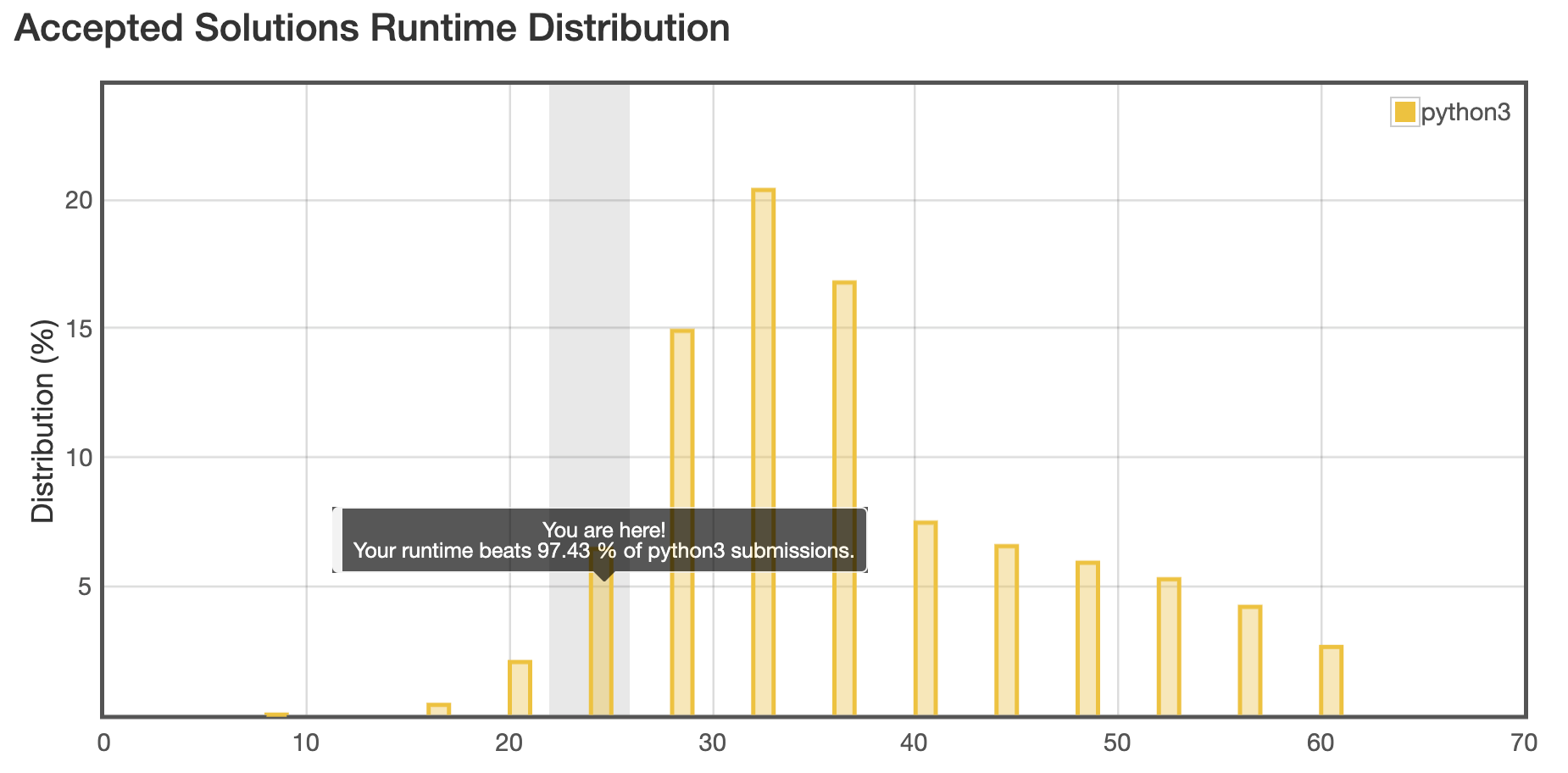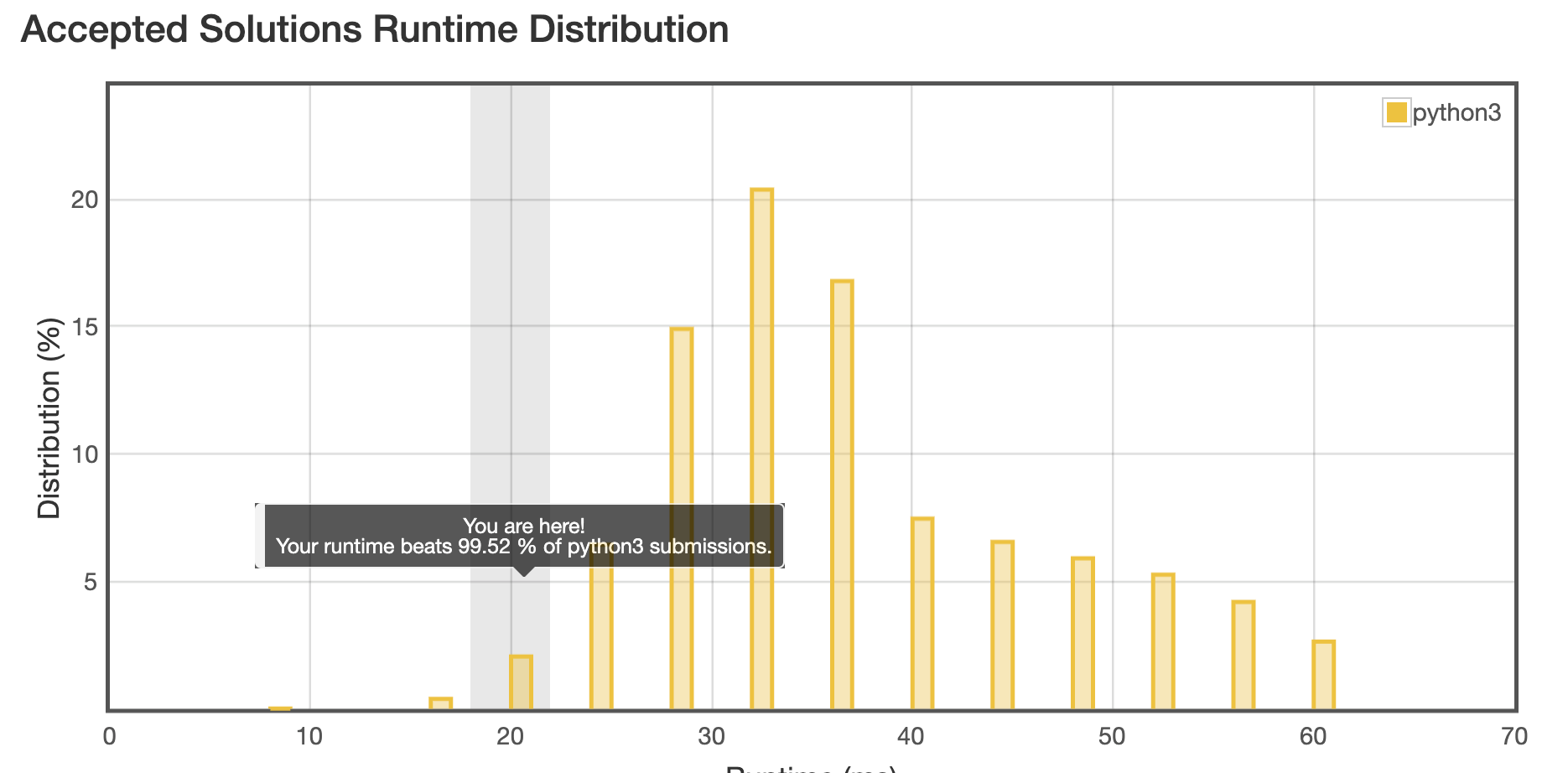# Longest Palindrome — Leetcode Challenge

Today’s Leetcode challenge was to figure out the maximum possible length a palindrome could be, given a string.

https://leetcode.com/explore/challenge/card/august-leetcoding-challenge/550/week-2-august-8th-august-14th/3423/

I started off by going right down a rabbit hole, and not even a CORRECT rabbit hole. I spent hours writing code to return the actual longest palindrome for the string, without changing the order of the characters in the string.

RTFI (Read the F*ing Instructions), right?

The challenge was to find the maximum POSSIBLE length, given ANY order of the given string. Not only did the challenge not want a string back, it didn’t care about the order of the string.

Totally different challenge.On the upside, the fact that it took me hours to solve what the challenge WASN’T, means I learned something besides RTFI. :) Some other day I’ll post the code I started out with, which answered that completely unasked question. For today, I’ll post the correct answer.

My first (correct) solution was 95% faster than other submissions. My new and improved second (correct) submission was 99+% faster, plus it used less memory. I’ll explain the logic, the first solution, and then why I changed what I did to create the second solution.

## Explanation of Challenge and Logic:

`Example Strings = “xxyyzzzzzzzaabcccdddde”`

A palindrome is a string that’s the same forwards and backwards. Find out the longest possible palindrome.

Each time there are 2 of the same characters in the string, that counts as 2 characters that could be arranged with others, to form a palindrome. For example, if the string is “aacc”, the palindrome could be “acca” or “caac”.

If, for example, there are 3 of the same characters, and two of another, one of the leftovers from the 3, could be the center of the palindrome. Once the center spot is taken, the odd leftovers aren’t counted for the rest of the string. This includes single instances of a character. For this string: “aaccddddbbb”, one palindrome could be “bdacdcadb”. The third “b” from the original string got discarded, because the “d” already had that center spot.

So the code should find the duplicate character count, and then if there’s a leftover from dividing one of the characters by 2, it should add a 1 (but only once) to the total number of characters.

`Example :“abccccdd” returns 7“cccc” = 4“dd” = 2 and either “a”, or “ b” can be the center, but not both.4 + 2 + 1 = 7Another Example:“aaaccccdd” returns 9“cccc” = 4 “dd” = 2 “aa” = 2There’s a leftover a, so “a” = 14 + 2 + 2 + 1 = 9`

Hopefully I’ve explained this in a way that makes sense but hasn’t put you to sleep.

Solutions and Explanations:

`Solution 1 - (Not counting the Rabbit Hole Solution for some imaginary problem I wasn't given))from collections import Counterdef longestPalindromeOne(s):    countDict = dict(Counter(s))    numChars = 0    center = False    for key, value in countDict.items():        divs = divmod(value, 2)        if divs > 0:            numChars += divs*2        if divs == 1 and center == False:            numChars += 1            center = True    return numChars`Solution 1 results

Solution 1 Explanation

The Counter module returns an iterable (an object that can be iterated through), that looks like this when printed:

`Counter({'c': 4, 'd': 2, 'a': 1, 'b': 1})`

After initializing center to False,I iterated through the Counter dictionary. (NOTE: I didn’t need to create a separate dictionary to do this. See Solution 2)

For each item in the dictionary, I used the built-in Python divmod function. divmod takes a value, and the amount to divide by, and returns a tuple with the result of the division, and the remainder. (divmod(5, 2) returns (2, 1)). I didn’t need to use this function, either, as you’ll see in Solution 2. So much learning.

Then I examine the first part of each tuple. If it’s a 0, then there is only one of that character. If it’s more than 1, the number is how many sets of 2 of that character there were. I re-multiplied the result by 2, (leaving out the remainder), and added that to the number of characters possible in the palindrome.

Then I examined the remainder. If a possible center hasn’t already been found, and the remainder is 1 on the character, 1 gets added the numChars and the center is set to True so another leftover doesn’t get added.

I should have switched around the center == False check, and the divs check, because it will likely fail more frequently on the center == False check, and therefore, the second part wouldn’t have been calculated. I’ll explain that more in a short bit.

Submission results for this solution: Even with the parts of this that weren’t ideal, the submission came in at 95+%. That’s not the worst result I’ve had. LOL.

Once I submit something, I don’t usually just leave it at that, though. I am there (and here in general) to learn, so I tinker.

First, I realized I didn’t need to create a dictionary, because I could iterate through the Counter result items. So, I took that out.

Then, I realized I didn’t actually need to know the remainder every time, so why bother using divmod? I changed that to integer divide the value by 2, which dumps the odd remainder. Then I re-multiplied that by 2, as before, to get the even number of characters that could go in the palindrome.

`Integer Division (//)5 // 2 * 2 = 4. 4 of the 5 characters can be used in a palindrome. 6 // 2 * 2 = 6, All 6 of that character can be used.`

Lastly, I swapped the if test on the center and the remainder calculation with each other. Now, if there’s an odd remainder, and the center is already set, I don’t need to execute the mod for any more numbers.

`original (still using divmod, and reversed):if divs == 1 and center == False:improved:if center == False and value % 2 == 1:`

Here’s why this matters
Iterating through “abbcddeffg”…

On ‘a’, the value of the center gets checked. On ‘a’, it’s False. Because of that, the mod is calculated for ‘a’. It comes back with 1, so center gets set to True.

When I get to “bb”, “c”, “dd”, etc…because the center is already set to True, there’s ZERO need to find out if the number of characters is odd or even.

If the first part of an if test fails, the second part is never executed. Look at the savings!

`Comparison for "abbcddeffg"original (still using divmod, and reversed)    if divs == 1 and center == Falseremainder test first, center test second11 comparisons7 remainder tests - once for each unique set of characters 4 center tests - once for each time there's a remainder (a, c, e, g)********improved:    if center == False and value % 2 == 1center test first8 comparisons7 center tests - once for each unique set of characters1 remainder test - once for the first character, and then it's set NOTE: If the center never gets set, then it could have done 7 center tests, and 7 remainder tests. This is where it's important to know your data.  What are the odds each one of those tests will pass onto the second test?  The one that's the most likely to stop execution of the second, is the one to put in the front.`

The impact of them ordering is minimal in this scenario, but, depending on what the tests do, it could have a significant performance impact.

`Solution 2 (My final for now. I've stopped tinkering to write this post)from collections import Counterdef longestPalindromeTwo(s):    numChars = 0    center = False    for key, value in Counter(s).items():        numChars += (value // 2) * 2        if center == False and value % 2 == 1:            center = True            numChars += 1    return numChars`Solution 2 results

I suspect there are solutions that use bitwise operations to solve the challenge, or any other number of possible solutions…as there ALWAYS are. For now, it’s time to stop tinkering with this one.

BUT, if you have an answer for this challenge you’d like to share? Please do, cuz I’m definitely here to learn.

Senior Software Engineer, Inventor, Writer, Zumba Instructor, Storm and Sky Photographer, Drone Pilot, Shar Pei Lover & Owner

## More from Binary Belle

Senior Software Engineer, Inventor, Writer, Zumba Instructor, Storm and Sky Photographer, Drone Pilot, Shar Pei Lover & Owner